Related Articles

# K-Means Clustering in Julia

• Last Updated : 12 Oct, 2020

Clustering is the task of grouping a set of objects(all values in a column) in such a way that objects in the same group are more similar to each other than to those in other groups. K-means clustering is one of the simplest and popular unsupervised machine learning algorithms.

Unsupervised algorithms make inferences from datasets using only input vectors without referring to known, or labeled, outcomes.

Define a target number k, which refers to the number of centroids you need in the dataset. A centroid is the imaginary or real location representing the center of the cluster.

Every data point is allocated to each of the clusters by reducing the in-cluster sum of squares. K-means algorithm identifies k number of centroids, and then allocates every data point to the nearest cluster while keeping the centroids as small as possible.

Note: Means in k-means refers to averaging of the data.

How it is achieved in Julia?

In Julia, it can be achieved with the help of some algorithms. K means is one such algorithm. This can simply be achieved with the help of inbuilt functions like kmeans().

This function divides the data into clusters having similar features: Data, no. of clusters we require, iter: keyword if we want to know how many iterations did it take to create clusters are passed as arguments in this function.

Packages involved:

• Dataframe
• Clustering
• Plots
• RDatasets

RDatasets package provides access to many of the classical data sets that are available in R.

## Julia

 `# syntax for loading the packages``using Pkg`` ` `# to create dataframes and load``Pkg.add(``"DataFrames"``)``using DataFrames`` ` `# to use the above function kmeans()``Pkg.add(``"Clustering"``)``using Clustering`` ` `# to visualise our clusters formed``Pkg.add(``"Plots"``)``using Plots`` ` `# RDatasets to load the already made datasets``Pkg.add(``"RDatasets"``)``using RDatasets`

Functions involved:

• dataset(): We can access Fisher’s iris data set using this function and passing the arguments as “datasets” and “iris”.
• Matrix(): Constructs an uninitialized Matrix{T} of size m×n.
• collect(): It returns an array of all items in the specified collection or iterator.
• scatter(): It is used to return scatter plot of dataset.
• rand(): Pick a random element or array of random elements from the set of values specified and dimensions and no of numbers is passed as an argument.
• nclusters(R): This function is used to match the number of clusters formed to the number of clusters we’ve passed in kmeans() function here R represents the results of returned clusters from kmeans().

#### Clustering in Existing Dataset

Following are the steps involved to perform clustering in Existing Dataset:

Step 1: In the dataset() function passing the datasets and iris as arguments and storing the data in the dataframe iris.

## Julia

 `# loading the data and storing it in iris dataframe``iris ``=` `dataset(``"datasets"``, ``"iris"``);`

Step 2: Now after storing the data in the dataframe we need to create a 2D Matrix which can be achieved with help of Matrix() function.

Step 3: Now storing the matrix in the features dataframe which represents the total rows and columns required to form a cluster of the fetched array with help of the collect() function.

## Julia

 `# Fetching the each value of data ``# using collect() function and ``# storing in features``features ``=` `collect(Matrix(iris[:, ``1``:``4``])');`

Step 4: Now applying the function kmeans() and passing the features and no. of clusters as arguments.

## Julia

 `# running  K-means for the 4 clusters``result ``=` `kmeans(features, ``4``);`

Step 5: Plotting the clusters with help of scatter() function to get scatter plot of 4 clusters here with different colors.

## Julia

 `# plot with the point color mapped ``# to the assigned cluster index``scatter(iris.PetalLength, iris.PetalWidth, ``        ``marker_z ``=` `result.assignments,``        ``color ``=``:blue, legend ``=` `false)`

Final Code:

## Julia

 `# loading the data and storing it in iris dataframe``iris ``=` `dataset(``"datasets"``, ``"iris"``);`` ` `# Fetching the each value of data ``# using collect() function and ``# storing in features``features ``=` `collect(Matrix(iris[:, ``1``:``4``])'); `` ` `# running  K-means for the 4 clusters``result ``=` `kmeans(features, ``4``);`` ` `# plot with the point color mapped``# to the assigned cluster index``scatter(iris.PetalLength, iris.PetalWidth,``        ``marker_z ``=` `result.assignments,``        ``color ``=``:blue, legend ``=` `false)`

Output: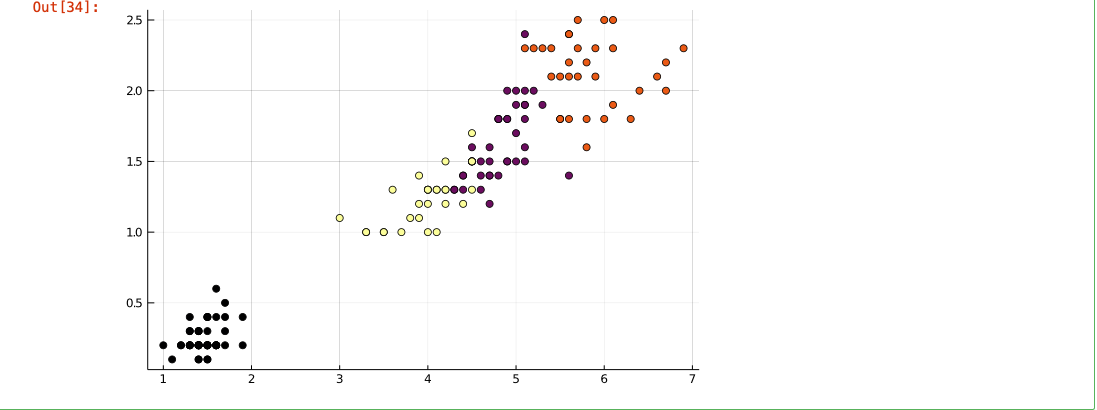#### Clustering after Creating a Dataset

Following are the steps involved to perform clustering after creating a Dataset:

Step 1: Forming a dataset with random numbers using rand() function and storing it into the dataframe also passing the dimensions required for the random numbers.

## Julia

 `# make a random dataset with ``# 1000 random 5-dimensional points``X ``=` `rand(``5``, ``1000``)`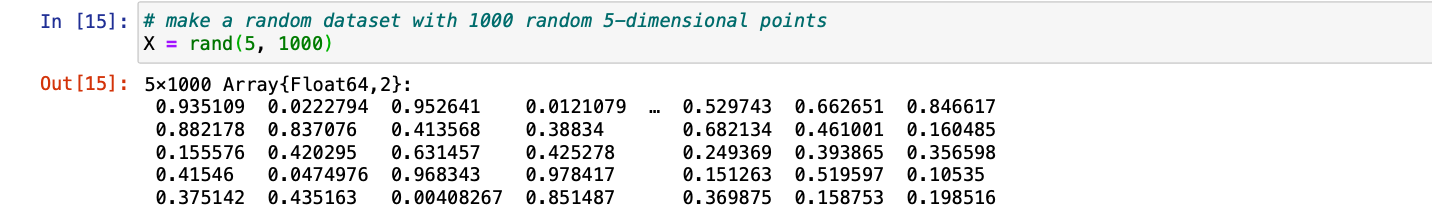Step 2: Now simply apply the kmeans() function and pass the random numbers dataframe, to display the iterations pass the argument display=:iter, also we need to pass the value for maxiter maximum iterations this function allowed to do.

## Julia

 `# cluster X into 20 clusters using K-means``R ``=` `kmeans(X, ``15``; maxiter ``=` `200``, ``                  ``display``=``:``iter``)`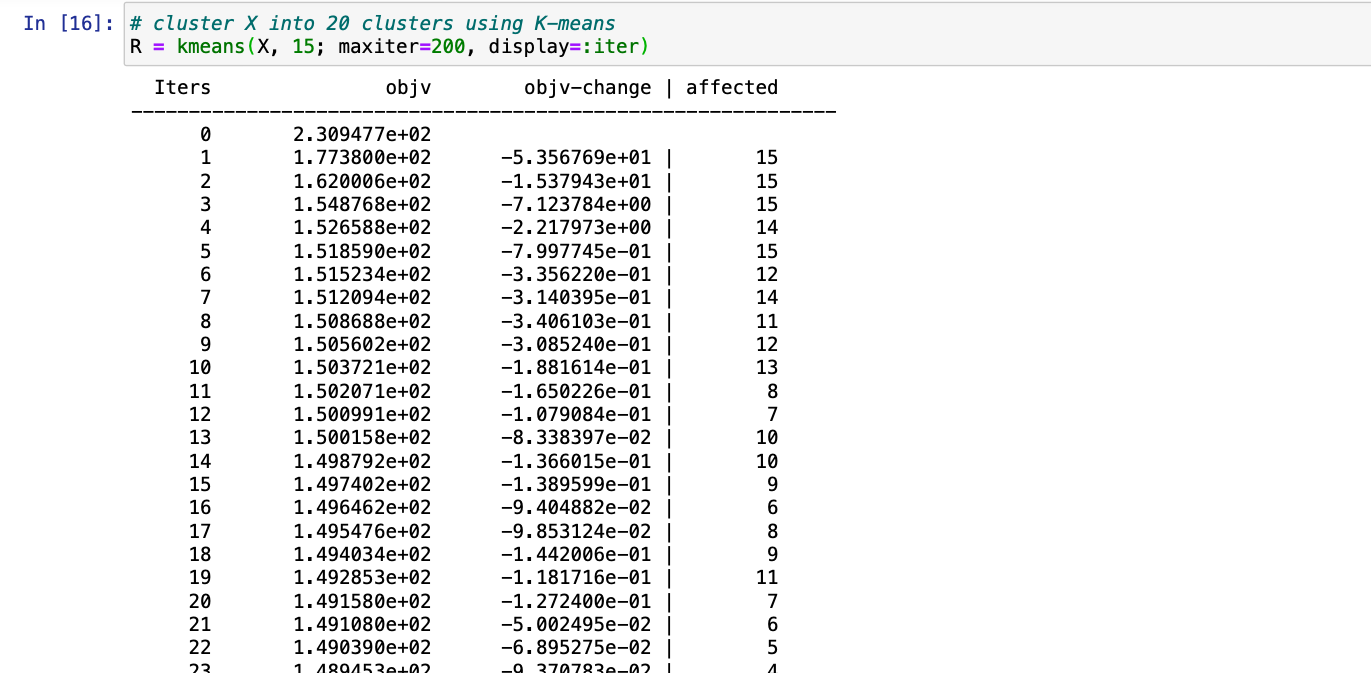Step 3: Verify the numbers of clusters formed with nclusters() function by passing the results of kmeans() as an argument, and we will get bool values true or false.

## Julia

 `# verify the number of clusters``nclusters(R) ``=``=` `15`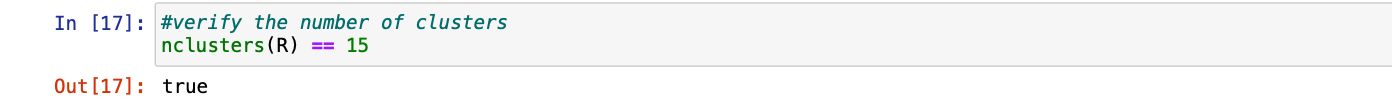Step 4: We can now get the assigned points to the clusters with help of assignments() function and passing the (R) returned clusters from kmeans().

## Julia

 `# get the assignments of points to clusters``a ``=` `assignments(R) `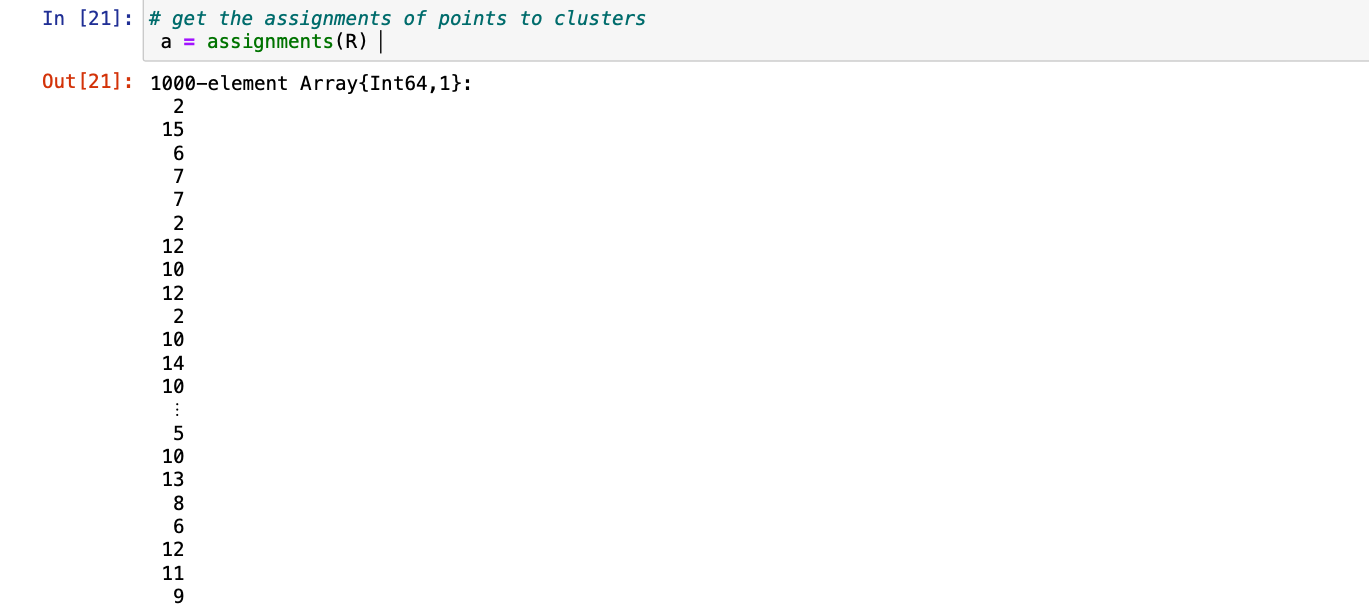Step 5: We can also get the sizes of the clusters with help of simple function counts(R)

## Julia

 `# get the cluster sizes``c ``=` `counts(R)`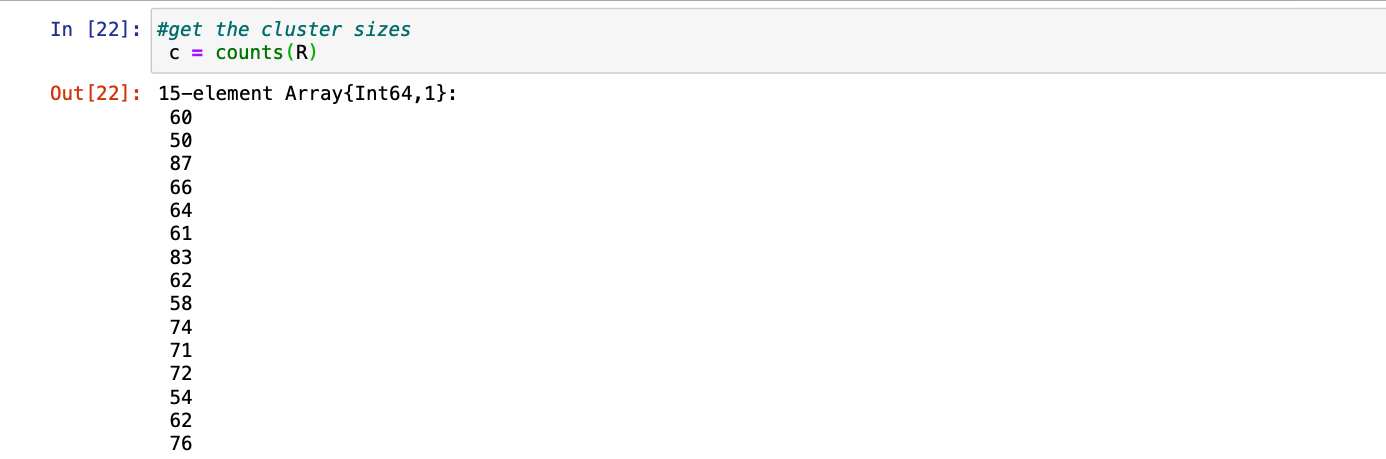Step 6: At last we can get values of cluster centers with R as object and accessing centers.

## Julia

 `# get the cluster centers``M ``=` `R.centers`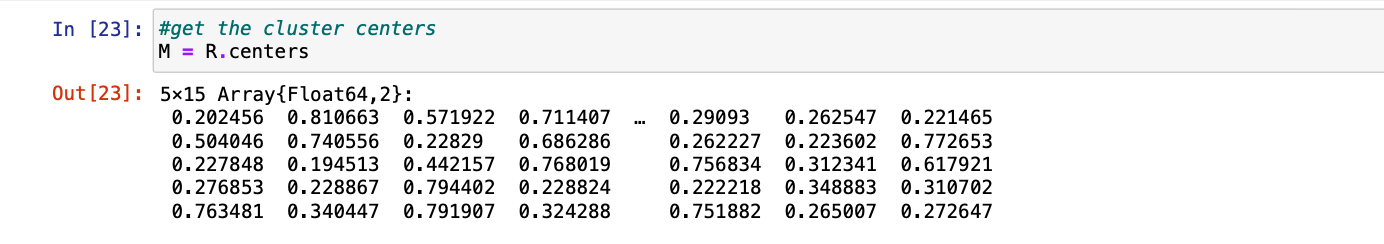Final Code:

## Julia

 `# make a random dataset with ``# 1000 random 5-dimensional points``X ``=` `rand(``5``, ``1000``)`` ` `# cluster X into 20 clusters using K-means``R ``=` `kmeans(X, ``15``; maxiter ``=` `200``, display``=``:``iter``)``  ` `# verify the number of clusters``nclusters(R) ``=``=` `15`` ` `# get the assignments of points to clusters``# a = assignments(R) `` ` `# get the cluster sizes``# c = counts(R)`` ` `# get the cluster centers``M ``=` `R.centers`

Output: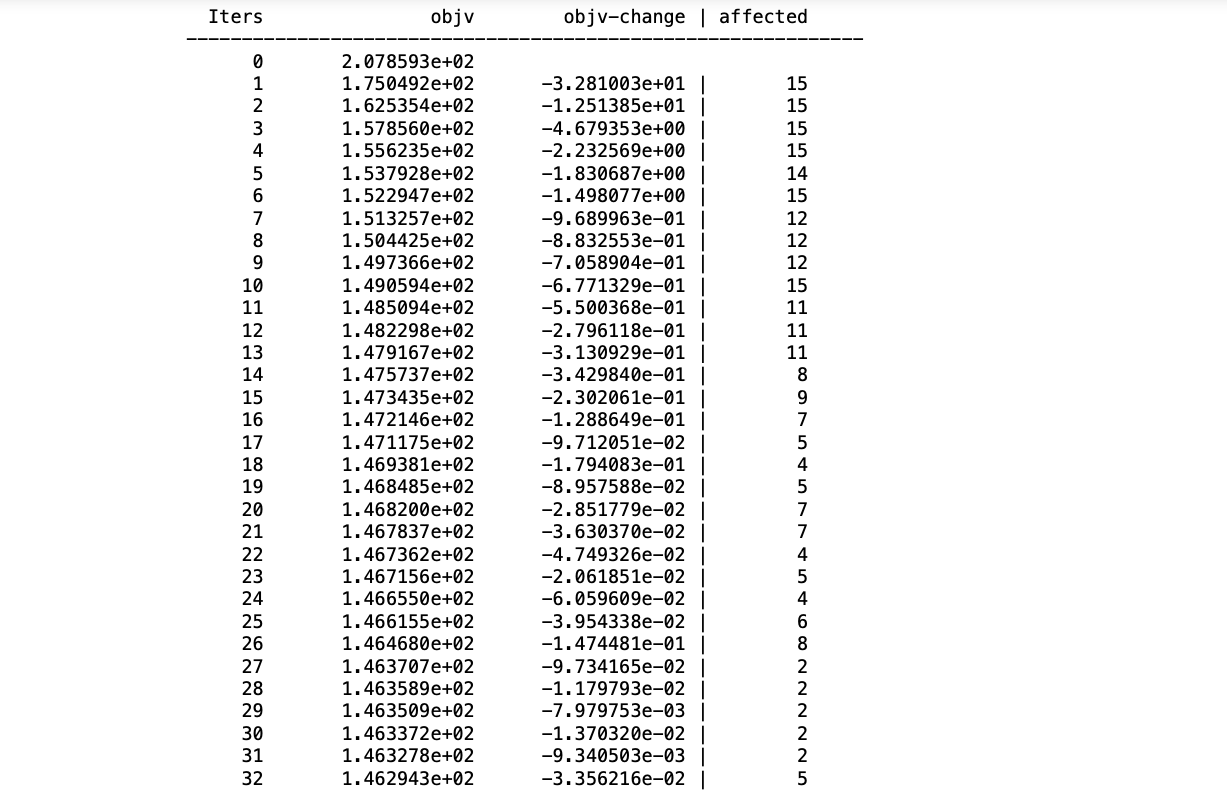My Personal Notes arrow_drop_up